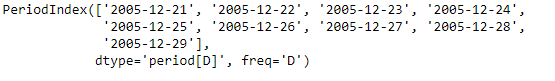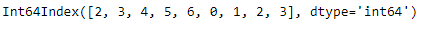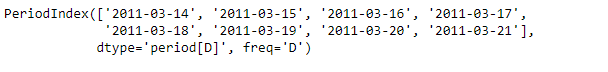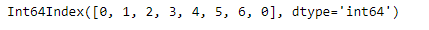# Python | Pandas PeriodIndex.dayofweek

Python is a great language for doing data analysis, primarily because of the fantastic ecosystem of data-centric python packages. Pandas is one of those packages and makes importing and analyzing data much easier.

Pandas` PeriodIndex.dayofweek `attribute returns an Index object containing the value of the days of the week for each element in the given PeriodIndex object. ‘0’ being the first day of the week and ‘6’ being the last day of the week.

Syntax : PeriodIndex.dayofweek

Parameters : None

Return : Index

Example #1: Use `PeriodIndex.dayofweek ` attribute to find the value of the days of the week for each element in the given PeriodIndex object.

 `# importing pandas as pd ` `import` `pandas as pd ` ` `  `# Create the PeriodIndex object ` `pidx ``=` `pd.PeriodIndex(start ``=``'2005-12-21'``,  ` `             ``end ``=``'2005-12-29'``, freq ``=``'D'``) ` ` `  `# Print the PeriodIndex object ` `print``(pidx) `

Output :Now we will use the `PeriodIndex.dayofweek` attribute to find the value of days of the week for each element in the given object.

 `# return the value of days of the week ` `pidx.dayofweek `

Output :As we can see in the output, the `PeriodIndex.dayofweek` attribute has returned an Index object containing the value of days of the week for each element in the given PeriodIndex obejct.

Example #2: Use `PeriodIndex.dayofweek` attribute to find the value of the days of the week for each element in the given PeriodIndex object.

 `# importing pandas as pd ` `import` `pandas as pd ` ` `  `# Create the PeriodIndex object ` `pidx ``=` `pd.PeriodIndex(start ``=``'2011-03-14 '``,  ` `              ``end ``=``'2011-03-21'``, freq ``=``'D'``) ` ` `  `# Print the PeriodIndex object ` `print``(pidx) `

Output :Now we will use the `PeriodIndex.dayofweek` attribute to find the value of days of the week for each element in the given object.

 `# return the value of days of the week ` `pidx.dayofweek `

Output :As we can see in the output, the `PeriodIndex.dayofweek` attribute has returned an Index object containing the value of days of the week for each element in the given PeriodIndex obejct.

My Personal Notes arrow_drop_upCheck out this Author's contributed articles.

If you like GeeksforGeeks and would like to contribute, you can also write an article using contribute.geeksforgeeks.org or mail your article to contribute@geeksforgeeks.org. See your article appearing on the GeeksforGeeks main page and help other Geeks.

Please Improve this article if you find anything incorrect by clicking on the "Improve Article" button below.

Article Tags :

Be the First to upvote.

Please write to us at contribute@geeksforgeeks.org to report any issue with the above content.